# Relation Between Median And Sides of a Triangle

Introduction To Median And Sides Of A Triangle

A triangle will have 3 medians. A line segment which joins any vertex of the triangle having the mid-point on its opposite side is called a median. It is also the line from the midpoint of a side to the opposite interior angle. They are concurrent at the centroid. The point which is common to all the 3 medians during their crossing is called a point of concurrency, the centroid of a triangle.

## How to Find the length of the Median

The different ways to find the length of a median are as follows:

• The formula for the length of the median to side BC =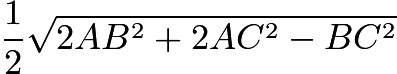.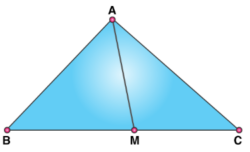• Using Apollonius’s Theorem, the formula for the median of a triangle is given by

m = $\sqrt{\frac{2b^2+2c^2-a^2}{4}}$

### Properties of Median of a Triangle

The properties of the median are as follows:

• The median bisects the vertex angle in an isosceles and equilateral triangle where the two adjacent sides are the same.
• The three medians of a triangle intersect at a point called the centroid.
• The area of the triangle is divided into half by a median.
• The triangle is divided into 6 smaller triangles of the same area by the centroid.
• In an equilateral triangle, the length of the medians is equal.
• The medians from the vertices having equal angles are of equal length in the case of an isosceles triangle.
• The length of the medians differs in a scalene triangle.
• The sum of two sides of a triangle is greater than the median drawn from the vertex, which is common.
• The median and lengths of sides are related in such a way that “3 times the sum of squares of the length of sides = 4 times the squares of medians of a triangle.”

3 (AB2 + BC2 + CA2) = 4 (AD2 + BE2 + CF2).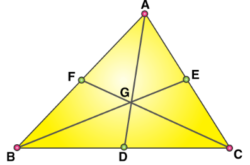• All the three medians intersect at one single point that divides the medians’ lengths in the ratio of 2:1.

## Solved Problems On Relation Between Median And Sides Of A Triangle

Example 1: In a ΔABC, \overline{AD}, \overline{BE} \and\ \overline {CF}\ are\ three\ medians.\ Then\ the\ ratio\ \overline {AD^2} + \overline {BE^2} + \overline {CF^2}\ : \overline {AB^2} + \overline {AC^2} + \overline {BC^2} is ___________.

Solution: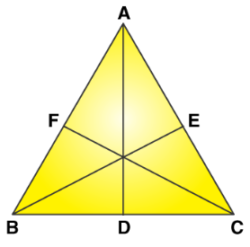In any triangle, the sum of the squares of any two sides is equal to twice the square of half of the third side together with twice the square of the median bisecting it.

∴ AB2 + AC2 = 2 (AD2 + BD2)

⇒ AB2 + AC2 = 2 (AD2 + BC2 / 4)

⇒ 2(AB2 + AC2 ) = 4 AD2 + BC2

Similarly, ⇒ 2 (AB2 + BC2) = 4 BE2 + AC2

⇒ 2(AC2 + BC2) = 4 CF2 + AB2

On adding all three 4 (AB2 + BC2 + AC2) = 4 (AD2 + BE2 + CF2) + BC2 + AC2 + AB2

⇒ 3 (AB2 + BC2 + AC2) = 4 (AD2 + BE2 + CF2)

⇒ [AD2 + BE2 + CF2] / [AB2 + BC2 + AC2]

= 3 / 4

Example 2: In the adjoining figure given, ∠PQR = 90 and QL is a median, PQ = 12cm, and QR = 14cm. Then, QL is equal to __________.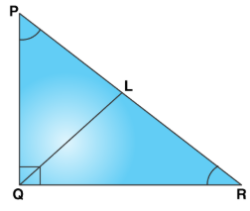Solution:

Given that, PQ = 5 cm, QR = 12 cm and QL is a median.

∴ PL = LR = PR2 …….(I)

In ΔPQR, (PR)2 = (PQ)2 + (QR)2 (By Pythagoras theorem)

= 144 + 256 = 400

⇒ PR = 20

Now, by theorem, if L is the mid-point of the hypotenuse PR of a right angled ΔPQR, then

QL = 1 / 2 [PR] = [1 / 2] * (20) = 10cm

Example 3: In a triangle, ABC, coordinates of A are (1, 2) and the equations of the medians through B and C are x + y = 5 and x = 4 respectively, then what is the area of ΔABC (in sq. units)?

Solution:

Median through C is x = 4

So, clearly the x coordinate of C is 4.

So, let C = (4, y), then the midpoint of A (1, 2) and C (2, y) which is D lies on the median through B by definition.

Clearly, D = ([1 + 4] / 2, [2 + y] / 2).

Now, we have, [3 + 4 + y] / 2 = 5 ⇒ y = 3. So, C = (4, 3).

The centroid of the triangle is the intersection of the medians.

It is easy to see that the medians x =4 and x + y = 5 intersect at G = (4, 1).

The area of triangle ΔABC = 3 × ΔAGC = 3 × 1 / 2 × 3 × 2 = 9.

(In this case, it is easy as the points G and C lie on the same vertical line x = 4. So the base GC = 2 and the altitude from A is 3 units.)

Example 4: If the median of ΔABC through A is perpendicular to AB, then find the relation between angles A and B.

Solution: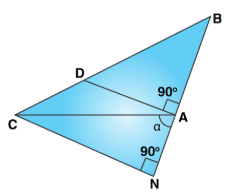We have BD = DC and ∠DAB = 90o.

Draw CN perpendicular to BA produced, then in ΔBCN, we have DA = 1 / 2 CN and AB = AN

Let ∠CAN = α [∵tanA = tan (π − α) = −tanα = −CN / NA =−2 [AD / AB] = −2 tanB

⇒ tan A + 2 tanB = 0.

Example 5: If a triangle PQR, sin P, sin Q, sin R are in A.P., then the altitudes are in _________.

Solution: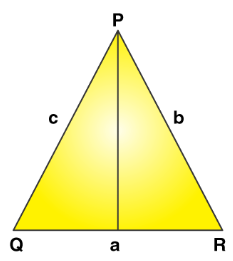PB = QC = l are in A.P. ⇒ a, b, c are in A.P.

∴ sinP / a = sinQ / b = sinR / c = λ

Let p1, p2, p3 be altitudes from P, Q, R.

p1 = c sinQ = λbc, p2 = a sinR = λac, p3 = b sinP = λab

Since a, b, c are in A.P.

Hence, 1 / a, 1 / b, 1 / c are in H.P.

⇒ abc / a, abc / b, abc / c are in H.P.

⇒ bc,ac,ab are in H.P.

⇒ λbc, λac, λab are in H.P.

⇒ p1, p2, p3 are in H.P.

Example 6: In the given figure below two medians AD and BE of ΔABC intersect at G at right angles. If AD = 18cm and BE = 12cm, then the length of BD (in cm) is _________.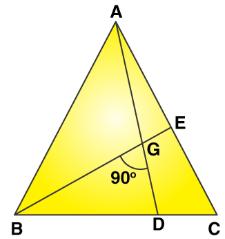Solution:

AD = 18cm. ⇒ GD = [1 / 3] × 18 = 6cm

BE = 12cm ⇒ BG = [2 / 3] × 12 = 8cm

∴ BD = √[62 + 82] = 10cm

Example 7: Let PS be the median of the triangle with vertices P (2, 2), Q (6, − 1)and R (7, 3). The equation of the line passing through (1, 1) and parallel to PS is _____________.

Solution:

S = midpoint of QR = ([6 + 7] / , [−1 + 3] / 2) = (13 / 2, 1)

PS = [2 − 1] / [2 − 13 / 2] = −2 / 9,

The required equation is y + 1 = −2 / 9 (x − 1)

i.e., 2x + 9y + 7 = 0